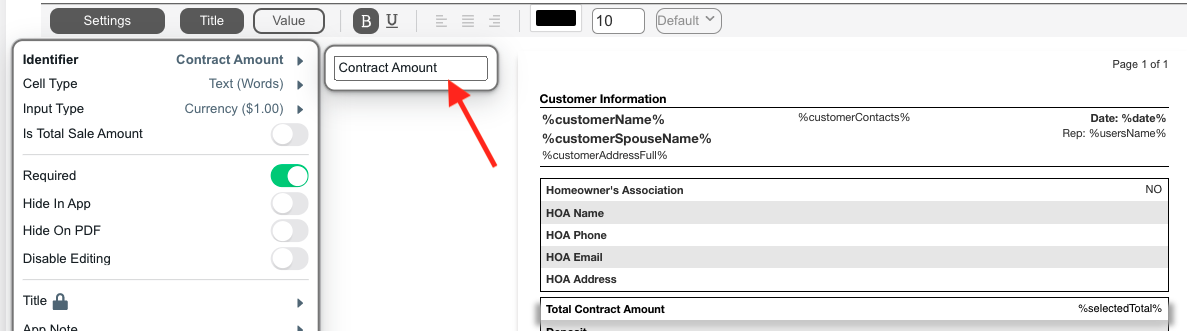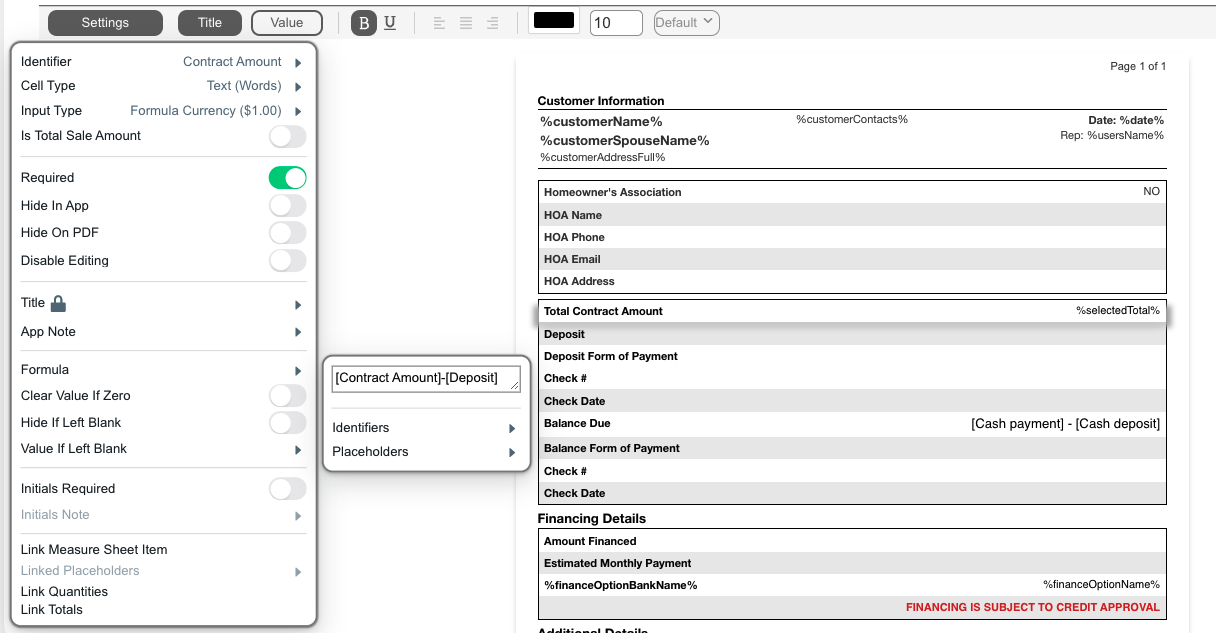# Formula Input Type

Formulas are used to perform mathematical functions within the Leap system in a document. There are four different formula input types. They all function the same except for the type of data output.
Formula (1)
Number without decimals.
Formula (1.00)
Number with decimals.
Formula (\$1)
Currency amount without decimals.
Formula (\$1.00)
Currency amount with decimals.
In the following example we will be subtracting the Deposit from the Contract Price in order to calculate the Balance Due On Completion.
1. In order to use Formulas the cells we are performing the formula on need Identifiers. Add an identifier of ‘Contract Amount’ and an identifier of ‘Deposit’ by clicking Settings on the cells.2. Next in the Balance Due On Completion cell change the Input Type to Formula Currency (\$1). In the Formula field use the Identifiers within square brackets to reference the values and subtract them. Enter the formula ‘[Contract Amount]-[Deposit]’.The following functions can be used in formulas to perform various operations.
Function Format/Syntax
Add  + 
Subtract  – 
Multiply  * 
Divide  / 
Raise (To Power)  ** 
Square Root sqrt()
Round Up ceiling()
Round Down floor()
Average average({, , , , , , })
Sum sum({, , , , , , })
Count count({, , , , , , })
Min min({, , , , , , })
Max max({, , , , , , })
Random Number randomn(10)